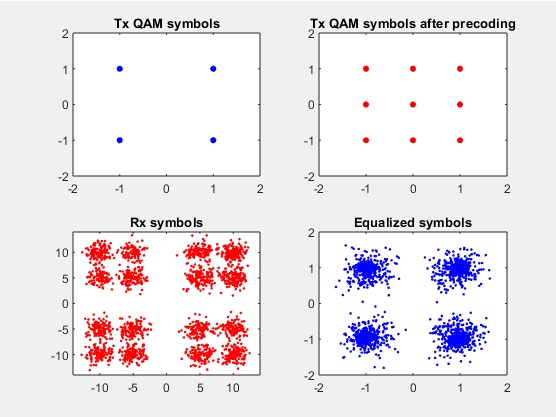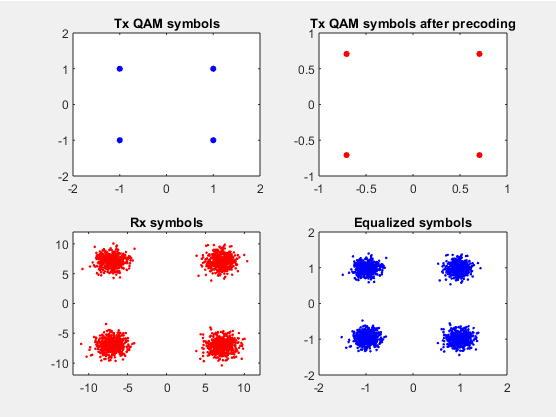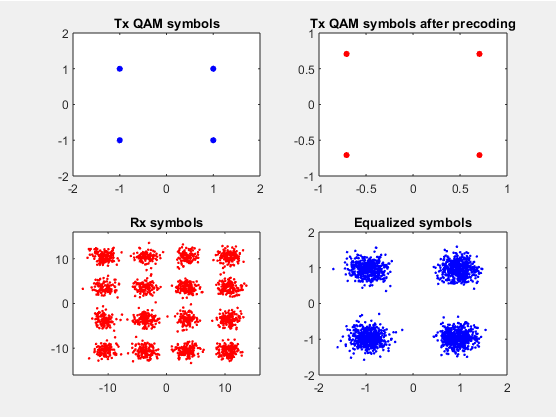Communication Technology

MMSE (Minimum Mean Square Error) - Matlab

This page is to show you some intuitive understandings about MMSE equalization. The original matlab code for this page is written by James Weng. I just modified it a little bit to get the type of plots that I want to get and change the function definition a little bit so that I can specify Precoding Matrix and Channel Matrix as parameters.

You may refer to MMSE page for deriving the mathematical representation of MMSE.

 Example 01 > Precoder = 1/2 * [1 1;1 -1]; Channel = [1 0;0 1]; Qam = 4; snr = 20;   fnDemoMmse(Qam,snr,Channel,Precoder);Example 02 > Precoder = 1/2 * [1 1;1 -1]; Channel = [1 0.5;0.5 1]; Qam = 4; snr = 20;   fnDemoMmse(Qam,snr,Channel,Precoder);Example 03 > Precoder = 1/sqrt(2) * [1 0;0 1]; Channel = [1 0;0 1]; Qam = 4; snr = 20;   fnDemoMmse(Qam,snr,Channel,Precoder);Example 04 > Precoder = 1/sqrt(2) * [1 0;0 1]; Channel = [1 0.5;0.5 1]; Qam = 4; snr = 20;   fnDemoMmse(Qam,snr,Channel,Precoder);Example 05 > Precoder = 1/2 * [1 1;1 -1]; Channel = [1 0.5;0.2 1]; Qam = 4; snr = 20;   fnDemoMmse(Qam,snr,Channel,Precoder);Example 06 > Precoder = 1/2 * [1 0;01]; Channel = [1 0.2;0.2 1]; Qam = 4; snr = 20;   fnDemoMmse(Qam,snr,Channel,Precoder);Example 07 > Precoder = 1/2 * [1 1;1 -1]; Channel = [1 0;0 1]; Qam = 16; snr = 20;   fnDemoMmse(Qam,snr,Channel,Precoder);Example 08 > Precoder = 1/2 * [1 1;1 -1]; Channel = [1 0;0 1]; Qam = 64; snr = 20;   fnDemoMmse(Qam,snr,Channel,Precoder);Example 09 > Precoder = 1/2 * [1 1;1 -1]; Channel = [1 0;0 1]; Qam = 64; snr = 30;   fnDemoMmse(Qam,snr,Channel,Precoder);fnDemoMmse.m function [dummy] = main(m_qam, snr_dB,H,P) % Demonstrate MMSE equalization % Inputs: %   m_qam:  QAM level. Square QAM only. %           Default = 16QAM. %   snr_dB: SNR per symbol in dB. %           Default = 20dB;   dummy = 0;   if (nargin < 1)    m_qam = 16; end if (nargin < 2)    snr_dB = 20; end   m_pam_i = floor(sqrt(m_qam + 1e-3)); % compute square root of QAM m_pam_q = m_qam / m_pam_i;   sig_amp = sqrt(10^(snr_dB/10));  % Signal amplitude   n_sym_per_layer= 1000;           % Number of symbols per layer to simulate   n_layers = 2;                    % Number of layers n_tx_ant = 2;                    % Number of Tx antennas n_rx_ant = 2;   rand('state', 3200);             % set random generator state   % Transmitted symbols with normalized power m_pwr = (m_qam - 1) / 3;         % average QAM power v_tx_sym = sqrt(1/m_pwr) * fnGenQam(m_pam_i, m_pam_q, n_layers, n_sym_per_layer);   v_tx_sym_precoded = P * v_tx_sym;  % symbols after precoding   % Received signal + noise (normalized power). v_noise = randn(n_rx_ant, n_sym_per_layer) + 1i * randn(n_rx_ant, n_sym_per_layer); v_R = sig_amp * H * v_tx_sym_precoded + v_noise;  % Each column is a vector of R for the received signal vector     % MMSE equalizer. Assuming sig_amp, H, and P are known G = sig_amp * H * P; I = eye(n_rx_ant); F = G' * inv(G * G' + I);   % Equalized symbols v_Y = F * v_R;   figure(101); subplot(2,2,1); plot(real(v_tx_sym), imag(v_tx_sym), 'bo','MarkerFaceColor',[0 0 1],'MarkerSize',4); iq_max = ceil( 1.1*max(real(reshape(v_tx_sym,1,[])))); axis([-iq_max iq_max -iq_max iq_max]); title('Tx QAM symbols');   subplot(2,2,2); plot(real(v_tx_sym_precoded), imag(v_tx_sym_precoded), 'ro','MarkerFaceColor',[1 0 0],'MarkerSize',4); iq_max = ceil( 1.1*max(real(reshape(v_tx_sym_precoded,1,[])))); axis([-iq_max iq_max -iq_max iq_max]); title('Tx QAM symbols after precoding');   subplot(2,2,3); plot(real(v_R), imag(v_R), 'r.'); iq_max = ceil( 1.1*max(real(reshape(v_R,1,[])))); axis([-iq_max iq_max -iq_max iq_max]); title('Rx symbols');   subplot(2,2,4); plot(real(v_Y), imag(v_Y), 'b.'); iq_max = ceil( 1.1*max(real(reshape(v_Y,1,[])))); axis([-iq_max iq_max -iq_max iq_max]); title('Equalized symbols');     % -------------------------------------------------------------------------------------- % Generate QAM symbols function v_qam = fnGenQam(pam_i, pam_q, nx, ny) v_qam= fnGenPam(pam_i, nx, ny) + 1i * fnGenPam(pam_q, nx, ny);   % Generate PAM symbols function v_pam = fnGenPam(pam, nx, ny) v_pam = rand(nx, ny) * pam; v_pam = 2 * ceil(v_pam) - 1 - pam;